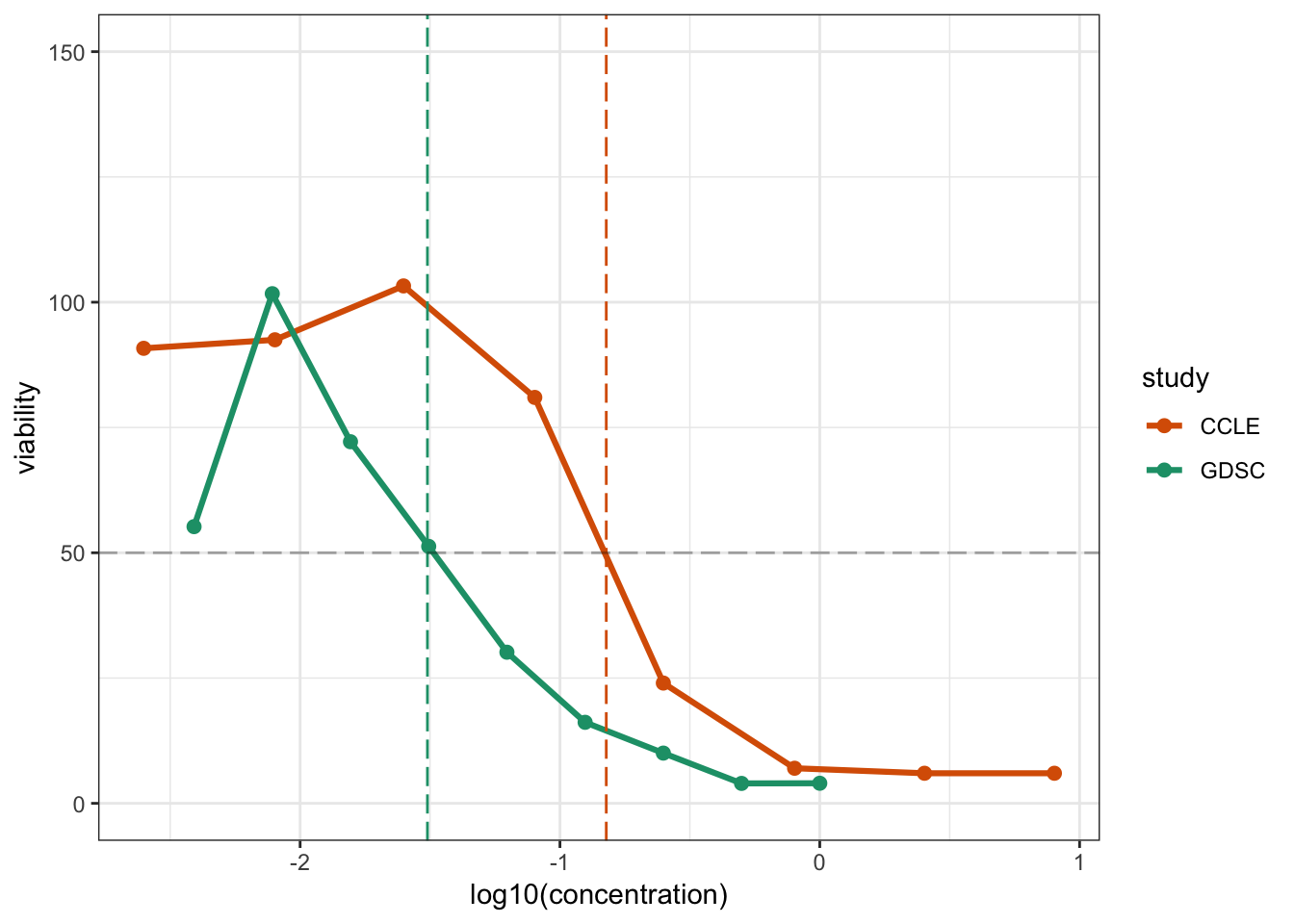## Introduction

IC50 and AUC statistics are designed to summarize drug response curves into a single number. This summarization step facilitates downstream analyses. Apart from summarizing drug responses, IC50 and AUC values also provide measures of the effect of drugs on cell lines. For an overview about these statistics, have a look at the Tutorial 1b (“Exploring Replicability with the `summarizedPharmacoData` Dataset”).

A limitation, however, of these types of summary statistics is that they usually require making assumptions about the data. As we will see in this tutorial, some of these assumption might not always hold. When going through this tutorial, try to think about the following question: Can the inconsistencies between the different studies be attributed to the modelling assumptions?

## Setup Workspace

We start by loading the tidyverse family of packages and specifying a default plotting theme for our `ggplot` graphics.

``library(tidyverse)``
``````## Registered S3 methods overwritten by 'ggplot2':
##   method         from
##   [.quosures     rlang
##   c.quosures     rlang
##   print.quosures rlang``````
``## ── Attaching packages ────────────────────────────────── tidyverse 1.2.1 ──``
``````## ✔ ggplot2 3.1.1     ✔ purrr   0.3.2
## ✔ tibble  2.1.2     ✔ dplyr   0.8.1
## ✔ tidyr   0.8.3     ✔ stringr 1.4.0
## ✔ readr   1.3.1     ✔ forcats 0.4.0``````
``````## ── Conflicts ───────────────────────────────────── tidyverse_conflicts() ──
``theme_set(theme_bw())``

We will be using both the raw and summarized pharmacological data in this tutorial.

``````pharmacoData <- readRDS(file.path("..", "data", "rawPharmacoData.rds"))

## Original Summaries

Let’s start by exploring the IC50 and the AUC statistics that were published in the original manuscripts. To do this, we’ll define a function, `plotResponse`, that allows us to visualize the relation between drug response and drug concentration. By writing a function to do the plotting, we reduce the amount of copying and pasting of code in our analysis (which can often introduce unexpected errors!). It also allows us to define a consistent way of plotting that can be applied to different subsets of the data.

``````plotResponse <- function(drugA, cellLineA) {
pharSub <- filter(pharmacoData, drug == drugA, cellLine == cellLineA)
sumSub <- filter(summarizedData, drug == drugA, cellLine == cellLineA)
ggplot(pharSub, aes(x = log10(concentration), y = viability, color = study)) +
geom_point(size = 2.1) +
geom_line(lwd = 1.1) +
ylim(0, 150) +
geom_vline(xintercept = log10(sumSub[,"ic50_CCLE"]),
color = "#d95f02", linetype = "longdash") +
geom_vline(xintercept = log10(sumSub[,"ic50_GDSC"]),
color = "#1b9e77", linetype = "longdash") +
geom_hline(yintercept = 50, col = "#00000050", linetype = "longdash") +
scale_colour_manual(values = c("CCLE" = "#d95f02", "GDSC" = "#1b9e77")) +
xlim(range(log10(c(pharSub\$concentration, sumSub\$ic50_CCLE, sumSub\$ic50_GDSC))))
}``````

The plot defined above will visualize the viability scores of a single cell line, `cellLineA`, for a single drug, `drugA`, as a function of the drug concentrations in each study. The vertical dotted lines display the IC50 value published from each study. Let’s start by exploring how the response curve for the drug `17-AAG` behaves in the cell-line `H4`. Notice that this drug was reported to have consistent viability responses between the two studies.

``plotResponse(drugA = "17-AAG", cellLineA = "H4")``What observations can you draw from this curve? Are the response data holding the assumptions to estimate an IC50 value?

Let’s now select another drug and cell line combination.

``plotResponse(drugA = "Nilotinib", cellLineA = "22RV1")``